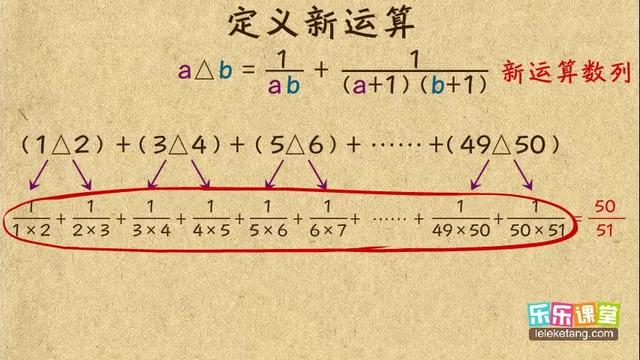# 定义新运算的知识点

## 1. 定义新运算

(1)理解新定义的算式含义；

(2)严格按照新定义的计算程序，将数值代入，将其转化为常规的加减乘除乘方运算，然后计算得结果.

1.定义一种新运算※，观察下列式子：

1※3=1*3+3=6;3※2=3*2+2=8;

3※5=3*5+5=20;5※3=5*3+3=18.

(1)填一填：2※4=________,a※b=________;

(2)请你依照上述运算方法，求（-3※7）※2的值.

2.定义一种关于“⊙”的新运算，观察下列式子：

1⊙3=1*4+3=7;

3⊙（-1）=3*4+(-1)=11;

5⊙4=5*4+4=24;

4⊙（-3）=4*4+(-3)=13.

(1)填空：5⊙（-6）=________;

(2)请你判断：当a≠b时，a⊙b______b⊙a（填“=”或“≠”），并说明理由.

3.用[x]表示不超过x的整数中的最大整数，例如：

[2.23]=2,[-3.24]=-4.计算下列各式：

(1)[3.5]+[-3]; (2)[-7.25]+[-].

4.在有理数的范围内，我们定义三个数之间的新运算“#”法则：a#b#c=.

(1)计算：4#(-2)#(-5)=________.(2)

## 2. 定义新运算是什么概念

（1）解决此类问题，关键是要正确理解新定义的算式含义，严格按照新定义的计算顺序，将数值代入算式中，再把它转化为一般的四则运算，然后进行计算。 （2）我们还要知道，这是一种人为的运算形式。

## 3. 有理数的运算有理数什么的听不懂······哪位可以帮我总结一下